### Home > A2C > Chapter 4 > Lesson 4.3.2 > Problem4-148

4-148.
1. Jessica's new dilemma is the following problem: Homework Help ✎

2. Use the idea of completing the square to write the following quadratic function in graphing form:

3. y = x2 − 6x −2.

4. As usual, Anita was looking over Jessica's shoulder as she read the problem. “You can't do that one with the tiles,” she announced.

5. “Why not?” asked Jessica.

6. “Because it has negatives in it, and our teacher said the tiles are only a good model for positive numbers, but not negatives,” Anita assured her.

7. “Who needs the tiles?” said Jessica. “I can use the idea of completing the square algebraically. See, the complete square for x2 − 6x is (x − 3)2 = x2 − 6x + 9 . So I just have to subtract 11 to get that expression to equal x2 − 6x − 2.”

1. Based on Jessica's method above, what is the graphing form of this equation? State the vertex and sketch the graph.

2. Use the idea of completing the square to write the function f (x) = x2 − 4x − 5 in graphing form. State the vertex and sketch the graph.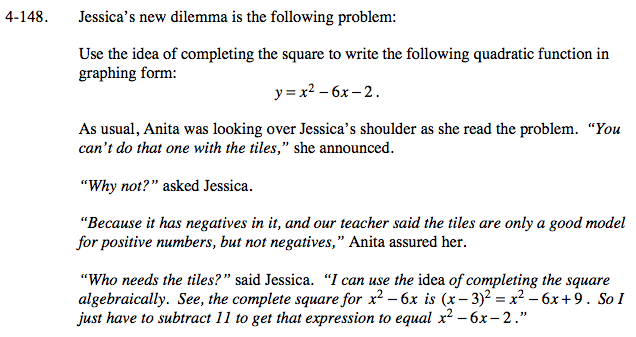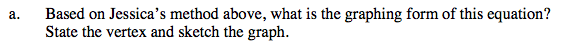y = x2 − 6x − 2

How many ones do you need to make a perfect square? How many do you have?

y = (x − 3)2 − 11
vertex = (3, −11)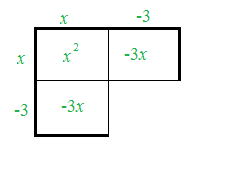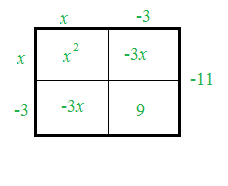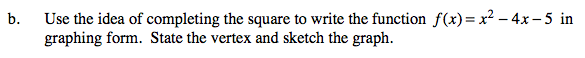See part (a).